# ENT 2493 ELECTRICAL CIRCUIT AND MACHINE 3 PHASE

• Slides: 41ENT 249/3 ELECTRICAL CIRCUIT AND MACHINE (3 -PHASE CIRCUITS)CONTENTS • INTRODUCTION • BALANCED THREE-PHASE VOLTAGES • BALANCED WYE-WYE CONNECTION • BALANCED WYE-DELTA CONNECTION • BALANCED DELTA-WYE CONNECTION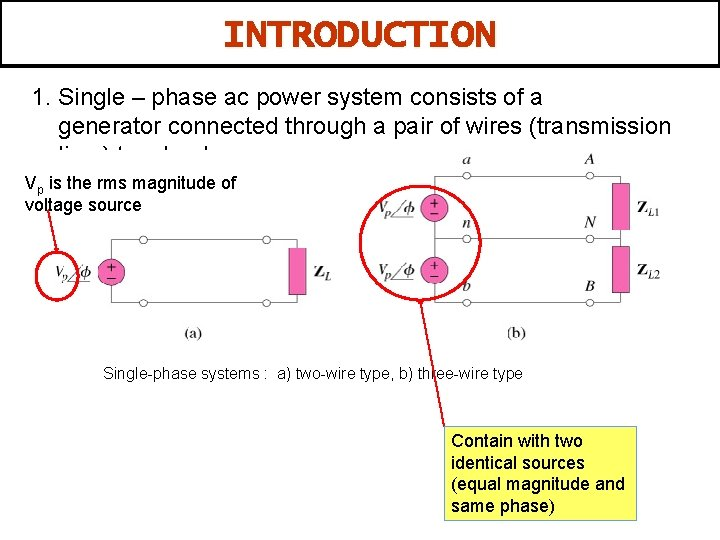INTRODUCTION 1. Single – phase ac power system consists of a generator connected through a pair of wires (transmission line ) to a load. Vp is the rms magnitude of voltage source Single-phase systems : a) two-wire type, b) three-wire type Contain with two identical sources (equal magnitude and same phase)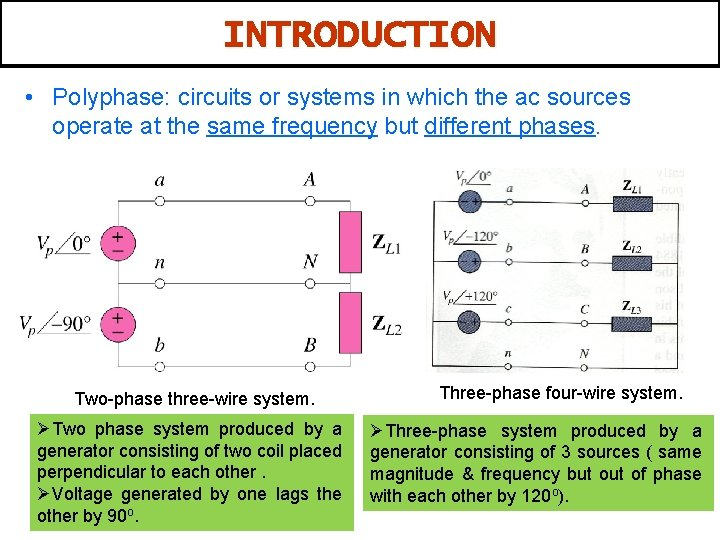INTRODUCTION • Polyphase: circuits or systems in which the ac sources operate at the same frequency but different phases. Two-phase three-wire system. ØTwo phase system produced by a generator consisting of two coil placed perpendicular to each other. ØVoltage generated by one lags the other by 90 o. Three-phase four-wire system. ØThree-phase system produced by a generator consisting of 3 sources ( same magnitude & frequency but of phase with each other by 120 o).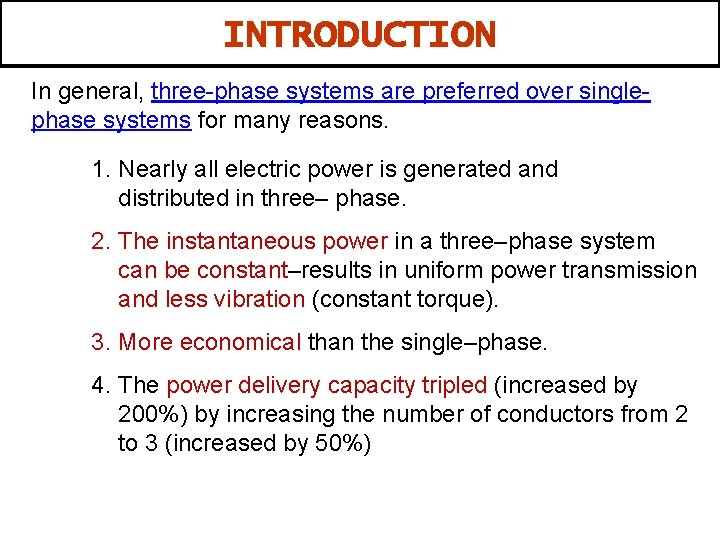INTRODUCTION In general, three-phase systems are preferred over singlephase systems for many reasons. 1. Nearly all electric power is generated and distributed in three– phase. 2. The instantaneous power in a three–phase system can be constant–results in uniform power transmission and less vibration (constant torque). 3. More economical than the single–phase. 4. The power delivery capacity tripled (increased by 200%) by increasing the number of conductors from 2 to 3 (increased by 50%)BALANCED THREE-PHASE VOLTAGES 1. The three-phase generator has three induction coils placed 120° apart on the stator. 2. The three coils have an equal number of turns, the voltage induced across each coil will have the same peak value, shape and frequency.BALANCED THREE-PHASE VOLTAGES 1. Three-phase voltages are often produced with a three– phase ac generator (or alternator) whose cross-sectional view as shown in Figure. A three-phase generator The generated voltages are 120 o apart from each other.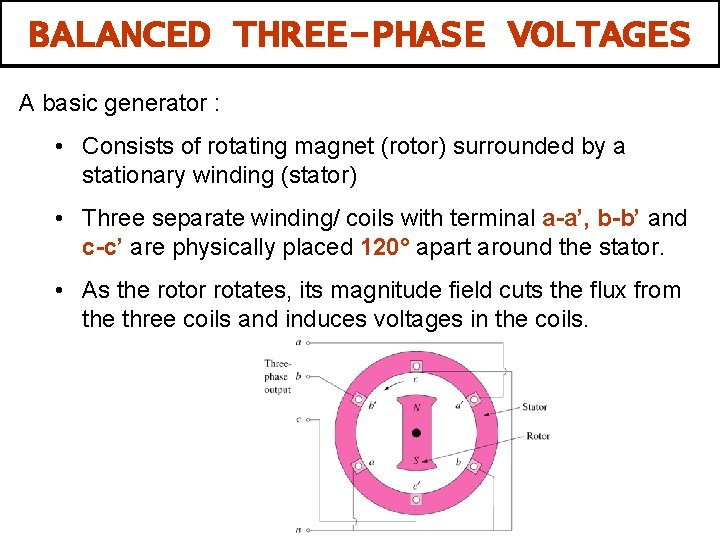BALANCED THREE-PHASE VOLTAGES A basic generator : • Consists of rotating magnet (rotor) surrounded by a stationary winding (stator) • Three separate winding/ coils with terminal a-a’, b-b’ and c-c’ are physically placed 120° apart around the stator. • As the rotor rotates, its magnitude field cuts the flux from the three coils and induces voltages in the coils.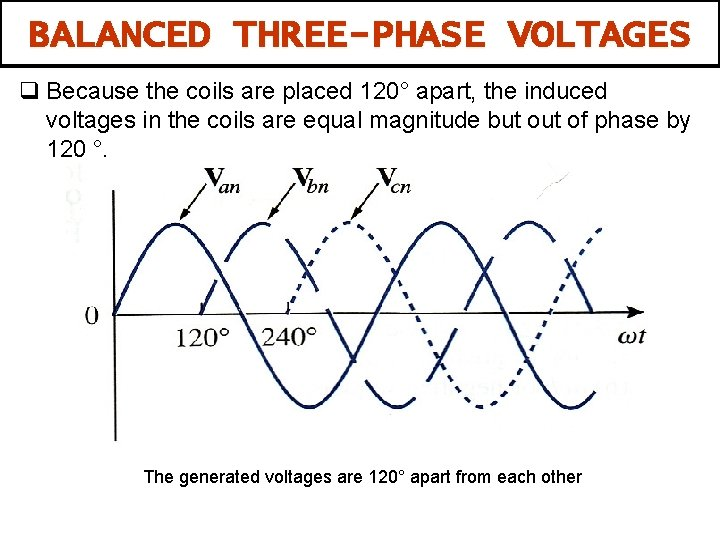BALANCED THREE-PHASE VOLTAGES q Because the coils are placed 120° apart, the induced voltages in the coils are equal magnitude but of phase by 120 °. The generated voltages are 120° apart from each other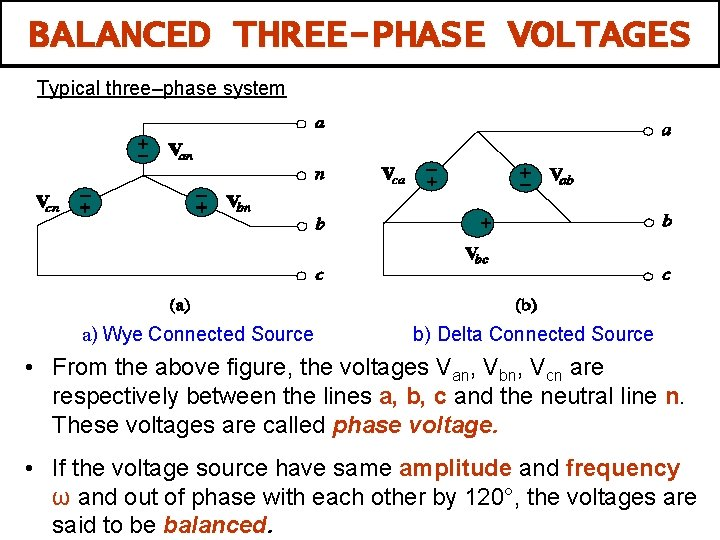BALANCED THREE-PHASE VOLTAGES Typical three–phase system a) Wye Connected Source b) Delta Connected Source • From the above figure, the voltages Van, Vbn, Vcn are respectively between the lines a, b, c and the neutral line n. These voltages are called phase voltage. • If the voltage source have same amplitude and frequency ω and out of phase with each other by 120°, the voltages are said to be balanced.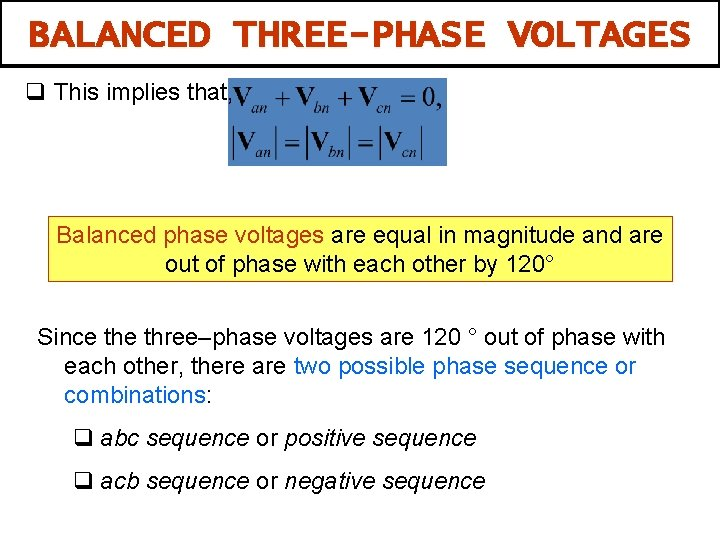BALANCED THREE-PHASE VOLTAGES q This implies that, Balanced phase voltages are equal in magnitude and are out of phase with each other by 120° Since three–phase voltages are 120 ° out of phase with each other, there are two possible phase sequence or combinations: q abc sequence or positive sequence q acb sequence or negative sequence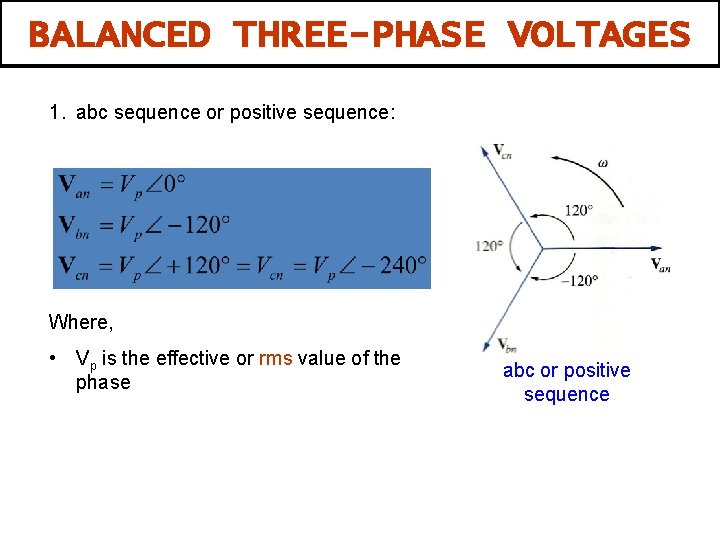BALANCED THREE-PHASE VOLTAGES 1. abc sequence or positive sequence: Where, • Vp is the effective or rms value of the phase abc or positive sequence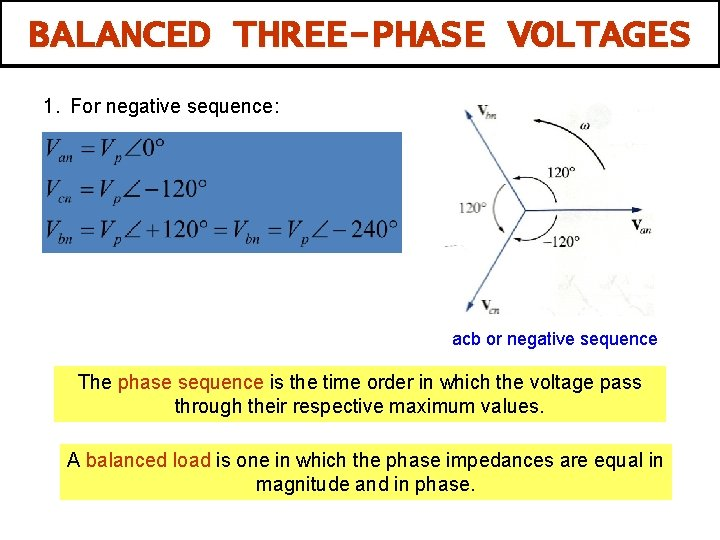BALANCED THREE-PHASE VOLTAGES 1. For negative sequence: acb or negative sequence The phase sequence is the time order in which the voltage pass through their respective maximum values. A balanced load is one in which the phase impedances are equal in magnitude and in phase.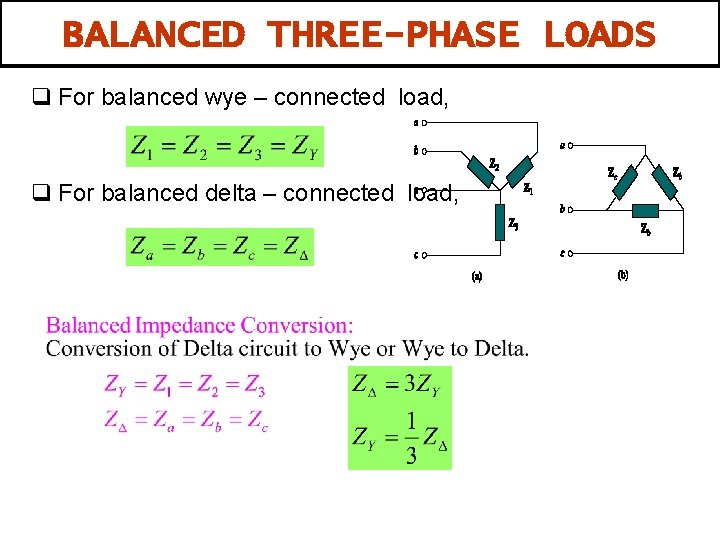BALANCED THREE-PHASE LOADS q For balanced wye – connected load, q For balanced delta – connected load,THREE-PHASE CONNECTIONS Ø Both the three phase source and the three phase load can be connected either Wye or DELTA. Four possibilities connections: • Wye – wye (Y-Y) connection • Wye – delta (Y-∆)connection • Delta – delta (∆ - ∆) connection • Delta – wye (∆ -Y) connection • Balanced Δ connected load is more common. • Y connected sources are more common.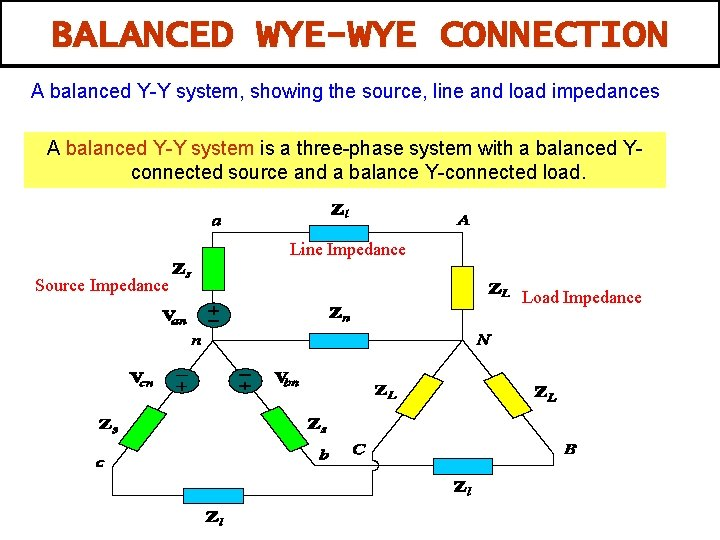BALANCED WYE-WYE CONNECTION A balanced Y-Y system, showing the source, line and load impedances A balanced Y-Y system is a three-phase system with a balanced Yconnected source and a balance Y-connected load. Line Impedance Source Impedance Load ImpedanceBALANCED WYE-WYE CONNECTION Four main elements in three–phase circuit: q Phase Voltage (VØ): measured between the neutral and any line , i. e line to neutral voltage. q Line Voltage (VL): measured between two of the three lines, i. e line to line voltage. q Line current (IL): current in each line of the source or load. q Phase current (IØ): current in each phase of the source or load.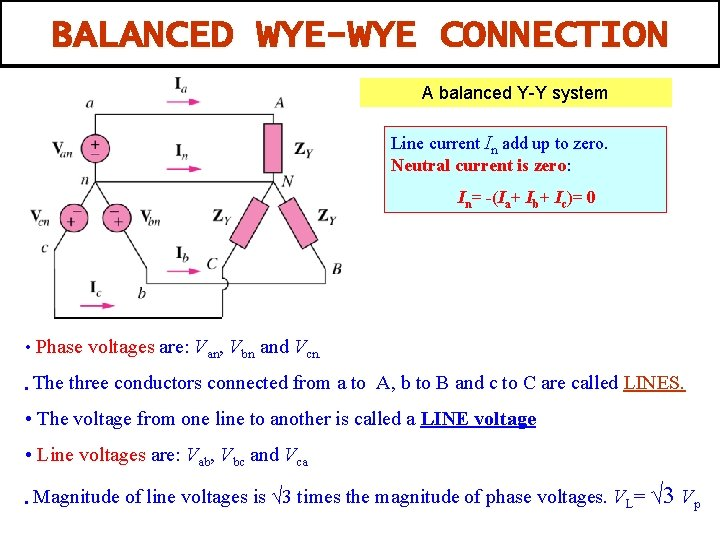BALANCED WYE-WYE CONNECTION A balanced Y-Y system Line current In add up to zero. Neutral current is zero: In= -(Ia+ Ib+ Ic)= 0 • Phase voltages are: Van, Vbn and Vcn. • The three conductors connected from a to A, b to B and c to C are called LINES. • The voltage from one line to another is called a LINE voltage • Line voltages are: Vab, Vbc and Vca • Magnitude of line voltages is √ 3 times the magnitude of phase voltages. VL= √ 3 Vp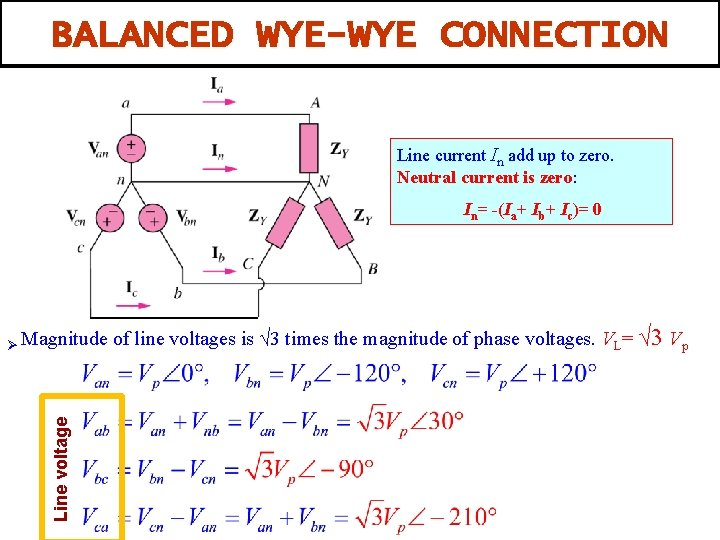BALANCED WYE-WYE CONNECTION Line current In add up to zero. Neutral current is zero: In= -(Ia+ Ib+ Ic)= 0 Line voltage Ø Magnitude of line voltages is √ 3 times the magnitude of phase voltages. VL= √ 3 Vp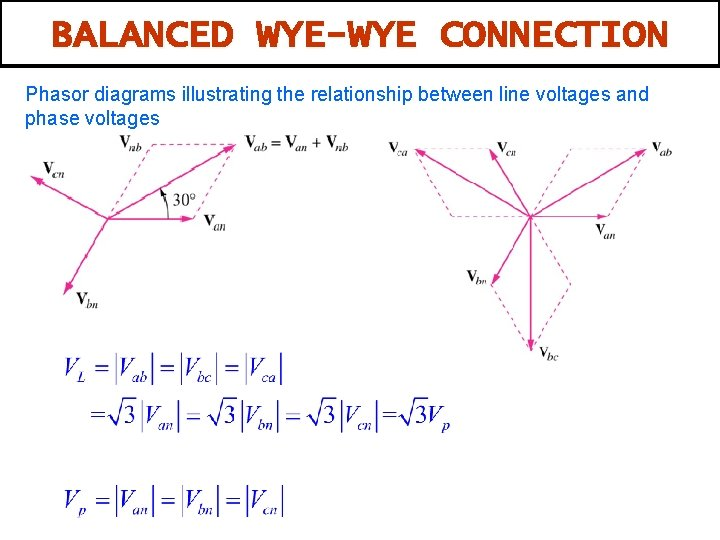BALANCED WYE-WYE CONNECTION Phasor diagrams illustrating the relationship between line voltages and phase voltagesSINGLE PHASE EQUIVALENT OF BALANCED Y-Y CONNECTION • Balanced three phase circuits can be analyzed on “per phase “ basis. . (single phase analysis) • We look at one phase, say phase a and analyze the single phase equivalent circuit. • Because the circuit is balanced, we can easily obtain other phase values using their phase relationships.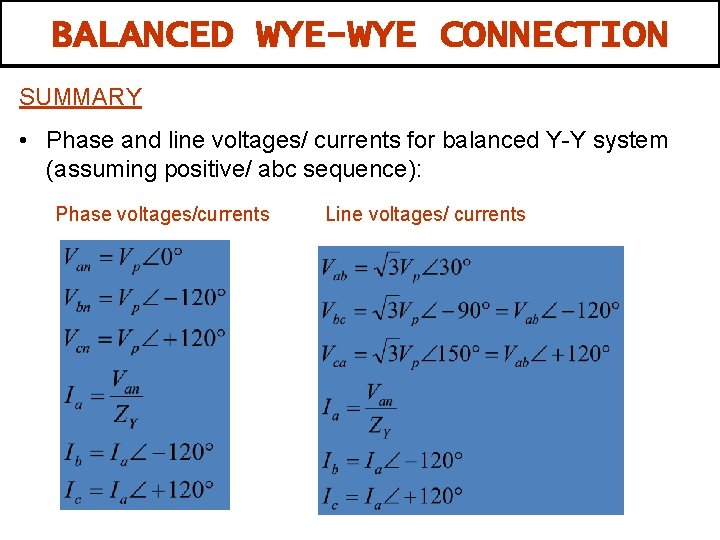BALANCED WYE-WYE CONNECTION SUMMARY • Phase and line voltages/ currents for balanced Y-Y system (assuming positive/ abc sequence): Phase voltages/currents Line voltages/ currentsEXAMPLE 1 A Y-connected balanced three-phase generator with an impedance of 0. 4+j 0. 3 Ω per phase is connected to a Yconnected balanced load with an impedance of 24+j 19 Ω per phase. The line joining the generator and the load has an impedance of 0. 6+j 0. 7 Ω per phase. Assuming a positive sequence for the source voltages and that Van=120 30° V. Find: 1. The line voltage 2. The line currentssolutionEXercise 1 A balanced three-phase generator has an abc phase sequence with phase voltage Van = 255∠ 0 o V. The generator feeds an induction motor which may represented by a balanced Y-connected load with an impedance of 12+j 5 Ω per phase. Assume a line impedance is 2Ω per phase. Calculate: 1. The line currents 2. The voltage for each loadBALANCED WYE-DELTA CONNECTION A balanced Y – ∆ system consists of a balanced Y-connected source feeding a balanced ∆ -connected load. • Three phase sources are usually Wye connected and three phase loads are Delta connected. • There is no neutral connection for the Y-∆ system. Phase current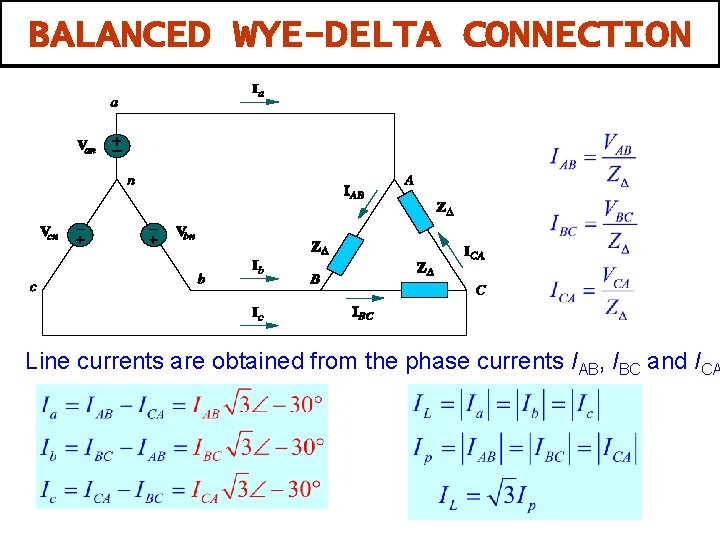BALANCED WYE-DELTA CONNECTION Line currents are obtained from the phase currents IAB, IBC and ICABALANCED WYE-DELTA CONNECTION • Assuming positive sequence, the phase voltage again: From previous section, the line voltage are The line voltages are equal to the voltages across the load impedances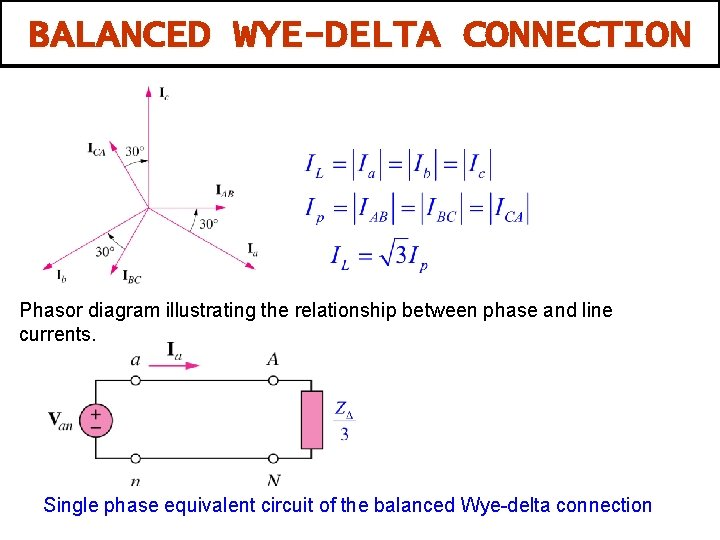BALANCED WYE-DELTA CONNECTION Phasor diagram illustrating the relationship between phase and line currents. Single phase equivalent circuit of the balanced Wye-delta connection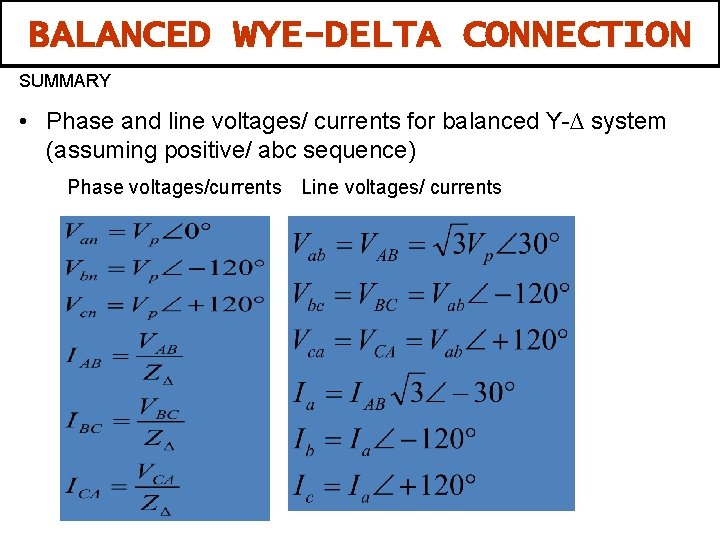BALANCED WYE-DELTA CONNECTION SUMMARY • Phase and line voltages/ currents for balanced Y-∆ system (assuming positive/ abc sequence) Phase voltages/currents Line voltages/ currents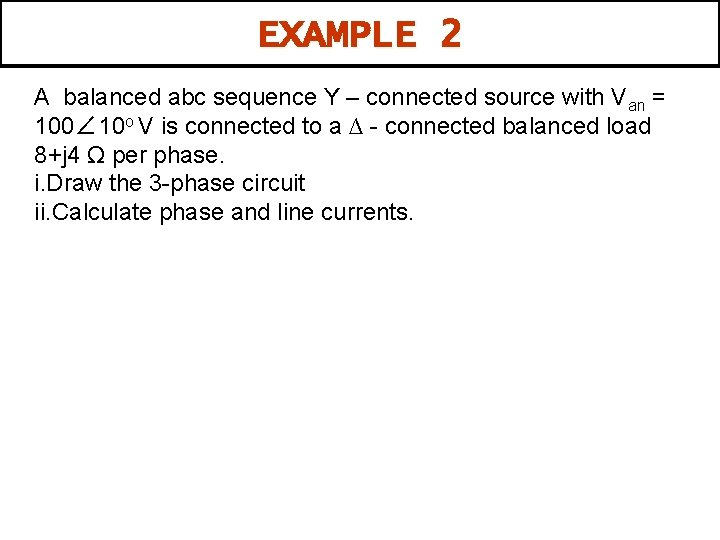EXAMPLE 2 A balanced abc sequence Y – connected source with Van = 100∠ 10 o V is connected to a ∆ - connected balanced load 8+j 4 Ω per phase. i. Draw the 3 -phase circuit ii. Calculate phase and line currents.BALANCED DELTA-DELTA CONNECTION A balanced ∆- ∆ system is one which both the balanced source and balanced load are ∆-connected. • Both the source and load are Delta connected and balanced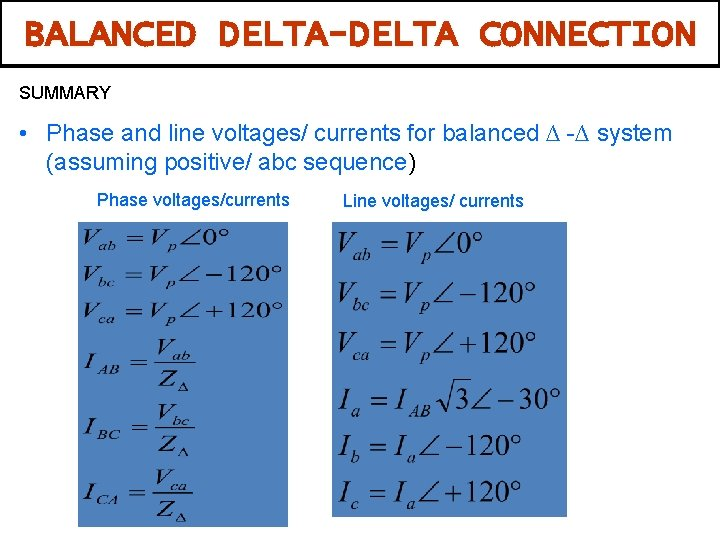BALANCED DELTA-DELTA CONNECTION SUMMARY • Phase and line voltages/ currents for balanced ∆ -∆ system (assuming positive/ abc sequence) Phase voltages/currents Line voltages/ currentsEXERCISE 2 One line voltage of a balance Y – connected source is VAB = 240 ∠-20 o V. if the source is connected to a ∆ - connection load of 20∠ 40 o Ω, Calculate the phase and line current. Assume the abc sequence.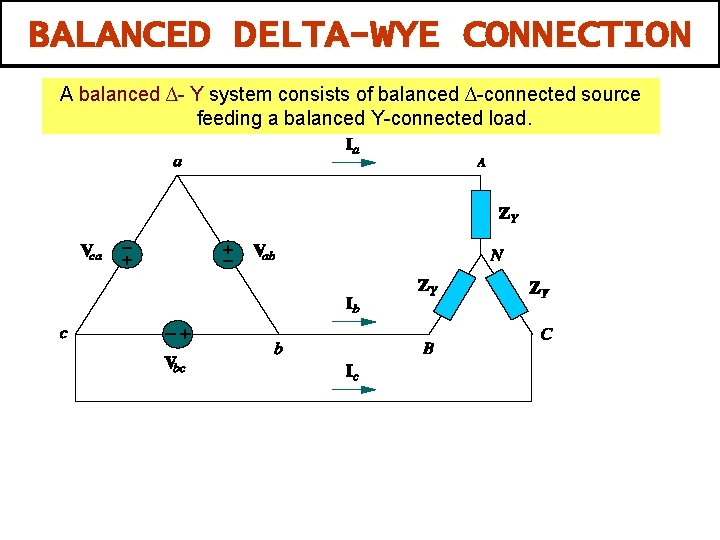BALANCED DELTA-WYE CONNECTION A balanced ∆- Y system consists of balanced ∆-connected source feeding a balanced Y-connected load.BALANCED DELTA-WYE CONNECTION Transforming a Delta connected source to an equivalent Wye connection Single phase equivalent of Delta Wye connection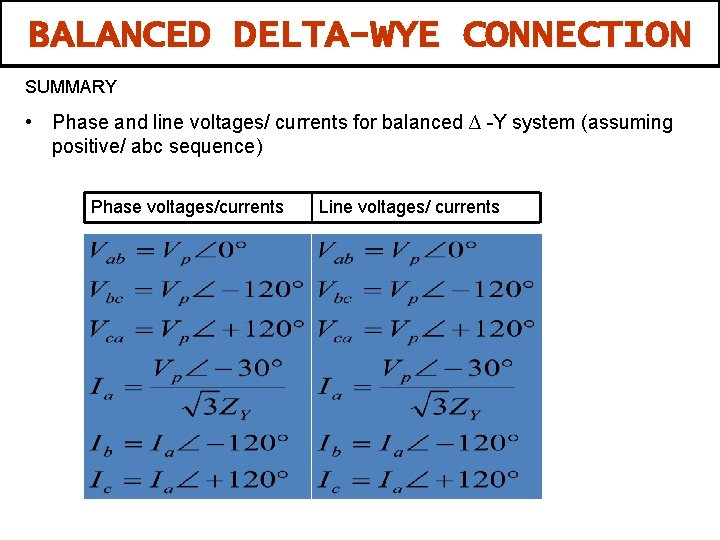BALANCED DELTA-WYE CONNECTION SUMMARY • Phase and line voltages/ currents for balanced ∆ -Y system (assuming positive/ abc sequence) Phase voltages/currents Line voltages/ currentsEXAMPLE 3 In a balance ∆-Y connected circuit, Vab=240 ∠ 15 o V and Zy= 12+j 15Ω. i. Draw the circuit ii. Calculate the line currents.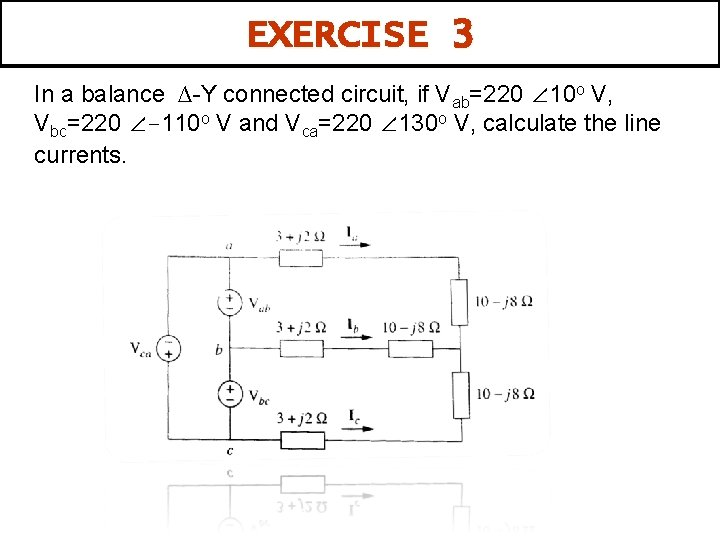EXERCISE 3 In a balance ∆-Y connected circuit, if Vab=220 ∠ 10 o V, Vbc=220 ∠-110 o V and Vca=220 ∠ 130 o V, calculate the line currents.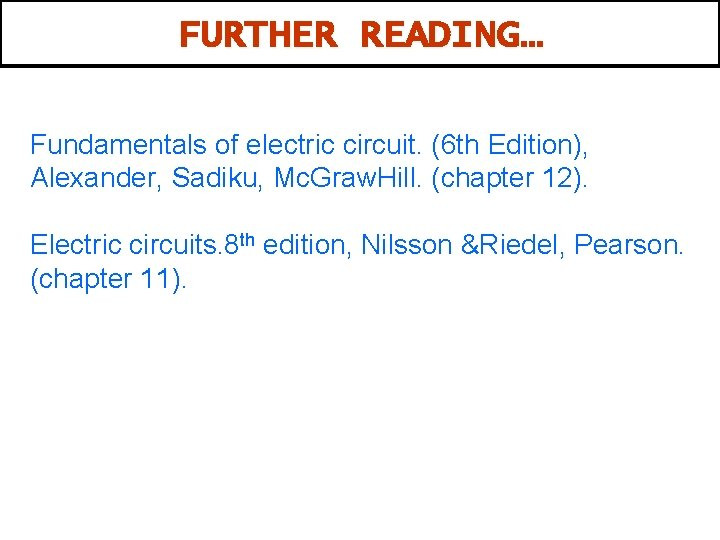FURTHER READING… Fundamentals of electric circuit. (6 th Edition), Alexander, Sadiku, Mc. Graw. Hill. (chapter 12). Electric circuits. 8 th edition, Nilsson &Riedel, Pearson. (chapter 11).